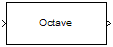# Octave Filter

Octave-band and fractional octave-band filter

• Library:
• Audio Toolbox / Filters

•## Description

The Octave Filter block performs octave-band or fractional octave-band filtering independently across each input channel. An octave-band is a frequency band where the highest frequency is twice the lowest frequency. Octave-band and fractional octave-band filters are commonly used to mimic how humans perceive loudness. Octave filters are best understood when viewed on a logarithmic scale, which models how the human ear weights the spectrum.

## Ports

### Input

expand all

• Matrix input –– Each column of the input is treated as an independent channel.

• 1-D vector input –– The input is treated as a single channel.

This port is unnamed unless you specify additional input ports.

Data Types: `single` | `double`

#### Dependencies

To enable this port, select Specify from input port for the Center frequency (Hz) parameter.

Data Types: `single` | `double`

### Output

expand all

The Octave Filter block outputs a signal with the same data type as the input signal. The size of the output depends on the size of the input:

• Matrix input –– The block outputs a matrix the same size and data type as the input signal.

• 1-D vector input –– The block outputs an N-by-1 matrix (column vector), where N is the number of elements in the 1-D vector.

Data Types: `single` | `double`

## Parameters

expand all

If a parameter is listed as tunable, then you can change its value during simulation.

Tunable: No

• The maximum center frequency is the value that causes the upper band edge to be equal to the Nyquist frequency, Fs/2. Frequencies above this value are saturated.

• The minimum center frequency is the value that causes the lower band edge to be equal to 1 Hz. Frequencies below this value are quantized to 1 Hz.

To specify Center frequency (Hz) from an input port, select Specify from input port for the parameter.

Tunable: Yes

Tunable: Yes

• off –– The Octave Filter block runs at the input sample rate.

• on –– The Octave Filter block runs at two times the input sample rate. Oversampling minimizes the frequency warping effects introduced by the bilinear transformation. An FIR halfband interpolator implements oversampling before octave filtering. A halfband decimator reduces the sample rate back the input sampling rate after octave filtering.

Tunable: No

When you select this parameter, the block inherits its sample rate from the input signal. When you clear this parameter, you specify the sample rate in Input sample rate (Hz).

Tunable: No

Tunable: Yes

#### Dependencies

To enable this parameter, clear the Inherit sample rate from input parameter.

• `Code generation` –– Simulate the model using generated C code. The first time you run a simulation, Simulink® generates C code for the block. The C code is reused for subsequent simulations as long as the model does not change. This option requires additional startup time, but the speed of the subsequent simulations is comparable to ```Interpreted execution```.

• `Interpreted execution` –– Simulate the model using the MATLAB® interpreter. This option shortens startup time but has a slower simulation speed than ```Code generation```. In this mode, you can debug the source code of the block.

Tunable: No

The mask attenuation limits are defined in the ANSI S1.11-2004 standard.

• If the mask is green, the design is compliant.

• If the mask is red, the design breaks compliance.

Tunable: Yes

A 2048-point FFT is used to calculate the magnitude response.

Tunable: Yes

## Block Characteristics

 Data Types `double` | `single` Direct Feedthrough `no` Multidimensional Signals `no` Variable-Size Signals `yes` Zero-Crossing Detection `no`

expand all

## Algorithms

expand all

 , Sophocles J. Introduction to Signal Processing. Englewood Cliffs, NJ: Prentice Hall, 2010.

 Acoustical Society of America. American National Standard Specification for Octave-Band and Fractional-Octave-Band Analog and Digital Filters: ANSI S1.11-2004. Melville, NY: Acoustical Society of America, 2009.

## SupportGet trial now